Impact Factor 3.367 | CiteScore 4.3
More on impact ›

# Frontiers in Physiology

## ORIGINAL RESEARCH article

Front. Physiol., 20 August 2012 | https://doi.org/10.3389/fphys.2012.00322

# Construction of calcium release sites in cardiac myocytes

• 1 Department of Muscle Cell Research, Institute of Molecular Physiology and Genetics, Slovak Academy of Sciences, Bratislava, Slovakia
• 2 Department of Biochemistry and Structural Biology, Institute of Molecular Biology, Slovak Academy of Sciences, Bratislava, Slovakia

Local character of calcium release in cardiac myocytes, as defined by confocal recordings of calcium sparks, implies independent activation of individual calcium release sites based on ryanodine receptor (RyR) channel recruitment. We constructed virtual calcium release sites (vCRSs) composed of a variable number of RyR channels distributed in clusters in accordance with the experimentally observed cluster size distribution. The vCRSs consisted either of a single virtual calcium release unit (vCRU), in which all clusters shared a common dyadic space, or of multiple virtual calcium release units (CRUs) containing one cluster each and having separate dyadic spaces. We explored the stochastic behavior of vCRSs to understand the activation and recruitment of RyRs during calcium sparks. RyRs were represented by the published allosteric gating model that included regulation by cytosolic Ca2+ and Mg2+. The interaction of Mg2+ with the RyR Ca2+-binding sites and the refractory period of vCRSs were optimized to accord with the experimentally observed calcium dependence of calcium spark frequency. The Mg2+-binding parameters of RyRs that provided the best description of spark frequency depended on the number of RyRs assembled in the vCRSs. Adequate inhibitory effect of Mg2+ on the calcium dependence of RyR open probability was achieved if the vCRSs contained at least three clusters. For the distribution of the number of open RyRs in evoked calcium sparks to correspond to the experimentally observed distribution of spark calcium release fluxes, at least three clusters had to share a common virtual CRU, in which ∼3 RyRs open to form an average spark. These results reconcile the small cluster size and stochastic placement of RyRs in the release sites with the estimates of the amount of RyR protein, volume density of calcium release sites, and the size of calcium release sites in rat cardiac myocytes.

## Introduction

Calcium sparks (Cheng et al., 1993), the elementary calcium signals of excitation-contraction coupling in cardiac myocytes, result from activation of ryanodine receptors (RyRs). RyRs channels are clustered at the junctional membrane of the dyadic sarcoplasmic reticulum, thus forming the calcium releasing units (Sun et al., 1995). Stimulation of cardiac myocytes results in synchronous activation of calcium sparks (Cannell et al., 1994) by a calcium influx through the voltage-dependent calcium channels (DHPRs). Sparks occur also in the absence of stimulation under diastolic conditions (Cheng et al., 1993). Such resting calcium sparks are thought to constitute a significant component of calcium leak from the sarcoplasmic reticulum that regulates SR calcium content (Lukyanenko et al., 2001; Maier et al., 2003).

The relationships between RyR activity and calcium spark properties were partially cleared only recently. Specifically, the average number of RyRs that open during the spark was estimated to 2.7–20 (Bridge et al., 1999; Wang et al., 2004; Shkryl et al., 2012), and the calcium dependence of spark frequency was shown to be in line with the calcium dependence of RyR open probability (Lukyanenko et al., 2000; Gusev and Niggli, 2008; Zahradnikova et al., 2010b). The generally accepted image of calcium release units (CRUs) involved large clusters of tightly packed RyRs (Franzini-Armstrong et al., 1999; Soeller et al., 2007). Recent data indicate that both the RyR packing density and the size of CRUs is much smaller (∼14 RyRs per cluster; Baddeley et al., 2009) and highly variable, with RyRs arranged into clusters and superclusters (Baddeley et al., 2009; Hayashi et al., 2009). Quantitative composition of the superclusters has not been determined. It is not known as well, whether adjacent clusters share the same dyadic space and the same terminal cisterna. The observed amount of RyR protein (Bers and Stiffel, 1993) and the density of calcium release sites (Chen-Izu et al., 2006) indicate about 70 RyRs per release site (Soeller et al., 2007; Asghari et al., 2012).

The properties of calcium sparks should be governed by the single channel properties of RyRs, the size of RyR clusters, and the arrangement of clusters into release sites. Previously we have shown (Zahradnikova et al., 2010b) that the relationship between cytosolic calcium concentration and the frequency of spontaneous calcium sparks can be fully explained by gating of single, independent RyRs. Quantitative modeling of these processes with a uniform cluster size of 182 RyRs provided parameters of RyR gating in a very good accordance with both, the experimentally determined gating properties of the RyRs, and the calcium dependence of the frequency of spontaneous calcium sparks. It turned out, however, that changes in the size of the CRUs had a strong effect on the simulated calcium spark frequency (Zahradnikova et al., 2010b). Here we explored how the organization of RyRs into small and highly variable clusters can be brought into accordance with RyR gating.

Calcium release flux during calcium sparks has been shown previously to have quantal amplitudes due to opening of only a few RyRs on electrical stimulation (Wang et al., 2004). Quantal distribution of the spark release flux amplitudes is compatible with the model of CRU based on the RyR activation controlled by Mg2+/Ca2+ competition at the RyR activation sites (Zahradnikova et al., 2010b). According to this CRU model, designed with 182 RyRs per CRU, the Mg2+ ions occupy 92% of the activation sites on RyR channels and prevent explosive RyR activation by calcium. The low rate of Mg2+ dissociation primes only a few RyRs in the CRU for opening during the spark. Quantal distribution of release flux was shown to be compatible with smaller clusters as well (Zahradnikova et al., 2010a); however, quantitative correspondence with experiments has not been tested.

In this work, the model of calcium spark (Zahradnikova et al., 2010b) has been extended and used to explore the consequences of variability of RyR clusters and their arrangement into CRUs and calcium release sites that emerged from the recent ultrastructural studies (Baddeley et al., 2009; Hayashi et al., 2009).

## Materials and Methods

### Construction of RyR Clusters, Virtual CRUs, and Virtual Calcium Release Sites

The size of the simulated RyR clusters was determined, as previously described (Zahradnikova et al., 2010a), by drawing random numbers from the bi-exponential size distribution (Baddeley et al., 2009) with the following parameters:

$pnRyR=0.113e-nRyR3.2+0.032e-nRyR20,$

where nRyR is the number of RyR channels in the cluster (cluster size), and p(nRyR) is the probability that a randomly chosen cluster will have a size nRyR.

A predefined number (1, 2, 3, 5, or 10) of individual RyR clusters was randomly combined into virtual calcium release units (vCRUs), which were considered to share a common dyadic space, i.e., the RyRs in a single vCRU sensed the same cytosolic and luminal environment (Figure 1Ab). One such vCRU was assumed to form a virtual calcium release site (vCRS). Alternatively, a predefined number of vCRUs (1, 2, 3, 5, or 10) consisting of a single cluster were combined into a vCRS. In this case, individual vCRUs constituting the vCRS were considered to behave independently, i.e., to have separate dyadic spaces (Figure 1Ac).

FIGURE 1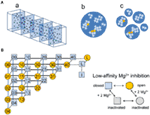Figure 1. Description of the model. (A) Construction of the model. (a) The simulated volume of a cardiomyocyte (10 μm × 3.16 μm × 3.16 μm), consisting of 5 sarcomeres, each comprising 20 calcium release sites observable by the confocal microscope (blue dots). (b) An example of a virtual calcium release site consisting of one virtual calcium release unit (blue circle) comprising five RyR clusters. (c) An example of a virtual calcium release site consisting of five virtual calcium release units (blue circles), each comprising one RyR cluster. Closed RyRs are light blue, open RyRs are orange. (B) The gating scheme of the RyR channel. According to this allosteric homotetrameric RyR model (Zahradnikova et al., 2010b), the single channel properties of RyR channels are described by Eq. 3 and the parameter set given in Table 2. The light blue squares represent the closed states and the orange circles represent the open states of the channel. The first and the second numeral denote the number of calcium and magnesium ions, respectively, bound to the RyR Ca2+ activation sites. The black (horizontal) and the blue (vertical) lines indicate the calcium-dependent and the magnesium-dependent channel transitions, respectively. The transitions independent of ion binding/unbinding are depicted as dashed lines. All transitions between channel states are reversible and obey the principle of detailed balance. L stays for the low activity L-mode states, and I stays for the inactivated state. Inactivation of the channel by Mg2+ ions binding to the low-affinity inhibition site is depicted at the right.

### Frequency of Spontaneous Calcium Sparks

The frequency of spontaneous calcium sparks, Fspark, was estimated according to Zahradnikova et al. (2010b):

where PO is RyR open probability, nvCRS is the number of vCRSs per 100 μm of a random longitudinal line scan, trd is the release duration, trp is the refractory period, τO is the RyR open time, and nRyR is the average number of RyRs present in the calcium release sites. The values of the parameters are given in Table 1.

TABLE 1

The steady-state RyR open probability, PO, was expressed by the aHTG model of RyR gating (Zahradnikova et al., 2010b), in which the transitions between states differing in the occupation of the activation sites are described by the scheme in Figure 1B, and the channel is additionally regulated by an independent Mg2+ inhibition site. Thus, the open probability of the RyR is:

$PO=( KCLKCL( [ Ca ]4fMg4KCa4(fCa4KO00+KOL) +4[ Ca ]fCa3fMgKCa3KMgKOL(fMgKMg+[ Mg ])3 +fCa4KCa4KOL(fMgKMg+[ Mg ])4 +4[ Ca ]3fCafMg3KCaKMg3KOL(fMgKMg+4[ Mg ]) +6[ Ca ]2fCa2fMg2KCa2KMg2KOL( fMg2KMg2+4fMgKMg[ Mg ] +[ Mg ]2 ) ) )/( [ Ca ]4fMg4KMg4( KCLKCIKOL +fCa4KO00( 1+KCI( 1+KCL+KCLKOL ) )) +4[ Ca ]fCa3fMgKCa3KCLKCIKMgKOL( fMg3KMg3(1+fCaKO00) +3fMg2KMg2(1+fCafMgKO00)[ Mg ] +3fMgKMg(1+fCafMg2KO00)[ Mg ]2 + (1+fCafMg3KO00)[ Mg ]3 ) +fCa4KCa4KCLKCIKOL( fMg4KMg4(1+KO00) +4fMg3KMg3(1+fMgKO00)[ Mg ] +6fMg2KMg2(1+fMg2KO00)[ Mg ]2 +4fMgKMg(1+fMg3KO00)[ Mg ]3+ (1+fMg4KO00)[ Mg ]4 ) +4[ Ca ]3fCafMg3KCaKCLKCIKMg3KOL( 2[ Mg ] +fMg (KMg+fCa3KMgKO00+fCa3KO00[ Mg ]) ) +6[ Ca ]2fCa2fMg2KCa2KCLKCIKMg2KOL4fMgKMg[ Mg ]+[ Mg ]2 +fMg2( KMg2(1+fCa2KO00)+2fCa2KMgKO00[ Mg ] + fCa2KO00[ Mg ]2 ) ) ).KI2/(KI2+[ Mg ]2),$

where [Ca] and [Mg] are the free cytosolic concentrations of Ca2+ and Mg2+, and the equilibrium constants Kx and allosteric factors fx are described and the values of the parameters are given in Table 2.

TABLE 2

Calcium spark frequency of a set of random RyR clusters was simulated using the formula

derived from Eq. 2 for non-uniform cluster sizes, where N is the number of simulated vCRSs (nRyR)i is the number of RyRs in the ith simulated vCRS and the other symbols were explained previously.

### The Number of Open RyRs

The distribution of the number of open RyRs in a virtual CRU was calculated using the binomial equation (Zahradnikova et al., 2010b):

$PnO=nRyR-1nO-1pAnO-1-1-pAnRyR-nO+1,$

where nRyR is the number of RyRs in the given virtual CRU, nO is the number of activated/open RyRs in the vCRU, and P(nO) is the probability that nO out of nRyR RyRs will be open during the spark.

The values of pA that optimized the distribution of the number of RyRs for the whole set of simulated CRUs were determined by minimizing the sum of squares of the differences between the predicted probabilities and those observed by Wang et al. (2004):

$χ2=∑i=1nPi-Pi2n-1,$

where i is the number of open RyRs, P(i) was determined by applying Eq. 4 to all vCRUs, Pi is the probability of occurrence of the ith quantal level, i.e., the number of open RyRs, determined by Wang et al. (2004), and n is the number of RyRs in the largest of the simulated vCRUs. The goodness of fit for each simulation was evaluated by the χ2 test (Press et al., 1992), using the method of Landau and Páez (1997) to determine experimental variance, as described previously (Zahradnikova et al., 1999).

### Parameters of Mg2+ unbinding

The parameters of Mg2+ unbinding from the activation site of the RyR channel were determined from activation probability, pA, as described in Zahradnikova et al. (2010b). Briefly, for each simulation, the dependence of the probability of Mg2+ dissociation (pdis) during the spark on pA was calculated from the steady-state open probabilities of all ionic forms of RyR, defined by the number of bound Mg2+ and Ca2+ ions, using the parameters of the aHTG model determined for the respective simulation. The value of pdis together with an estimate of the time to the peak release flux (TTP) then yielded an estimate of Mg2+ dissociation rate, $kdisMg$, as described previously (Zahradnikova et al., 2010b):

$kdisMg=-ln1-pdisTTP,$

where all parameters have already been explained.

Derivation of equations was performed in Mathematica (Ver. 8.0, Wolfram Research). Analyses were performed in Mathematica (Ver. 8.0, Wolfram Research) and Origin (Ver. 8.0 SR6, OriginLab).

## Results

### Construction of the Model

A model of a segment of a cardiac myocyte, containing 100 calcium release sites (Figure 1Aa) was designed as follows: A set of 1000 RyR clusters containing altogether 14095 RyRs was generated by randomly drawing 1000 numbers from a bi-exponential distribution of cluster sizes (Eq. 1). The resulting set of random “RyR clusters” was randomly recombined into two sets of vCRSs with the same size distribution. In the first set, each vCRS contained a single supercluster CRU, into which the RyR clusters were combined, as depicted in Figure 1Ab. In the second set, the vCRS contained RyR clusters of the same size, each cluster forming a separate single-cluster CRU (Figure 1Ac).

The size distribution of the vCRSs containing 1, 2, 3, 5, or 10 clusters is shown in Figure 2 and quantitatively characterized in Table 3. For vCRSs composed of a single cluster, the size distribution was in a good agreement with the experimental data (Baddeley et al., 2009). In multi-cluster vCRSs, the minimum number of RyRs ranged from 1 to 51 and their maximum number ranged from 180 to 271, respectively, while the mean size ranged from 14 to 141 RyRs and the median size ranged from 7 to 136.5 RyRs (Table 3). With increasing number of clusters per vCRS, the size distribution of the vCRSs gradually changed from bi-exponential to nearly Gaussian. With increasing number of clusters per vCRS, the total number of vCRSs decreased (from 1000 to 100), which was reflected in the increased variance in the size distribution histograms. When the total number of the generated vCRSs was more than 100, i.e., for nclust < 10, a set of 100 vCRSs was randomly selected for further simulation.

FIGURE 2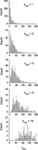Figure 2. Simulation of RyR distribution into clusters and virtual calcium release units (vCRUs). Distribution of the size of release units (in number of RyRs per release unit, nRyR) composed of (top to bottom) 1, 2, 3, 5, and 10 random clusters. The number of RyRs in clusters was distributed bi-exponentially according to Baddeley et al. (2009).

TABLE 3

In all models it was assumed that all RyRs in a CRU are equivalent in the sense that they share the cytosolic as well as the luminal environment, and therefore they have the same steady-state open probability, pO, and the same probability of being activated during a spark, pA. In other words, the cytosolic and luminal environments of the RyR channels in a single CRU were considered as ideally mixed compartments. The vCRSs with multiple CRUs (Figure 1Ac) emulate the effect of insufficient spatial resolution of confocal microscopes. The probability of RyR activation in any CRU was not affected by the activation of RyRs in the remaining CRUs. Individual vCRSs were fully independent as well.

### Calcium Dependence of Spark Frequency

The model of calcium spark frequency is based on the idea of triggering of a spark by the first spontaneous opening of single RyR channel in the calcium release site, arising from the resting open probability of RyRs (Zahradnikova et al., 2010b). In this model, all RyR channels in the vCRS are under resting conditions before activation of a calcium spark. The activation of a calcium spark corresponds to the stochastic opening of the first RyR in the vCRS. As a result, the frequency of sparks depends only on the total number of RyRs in the vCRS (Eq. 2) and not on the number and composition of vCRUs within the vCRS.

To adjust the constructed segments to real myocytes, we tuned their calcium dependence of calcium spark frequency to that measured by Lukyanenko and Gyorke (1999). The experimental calcium dependence of spark frequency (open symbols, Figure 3) was approximated by Eq. 2, into which Eq. 3 was substituted for PO. To obtain the best approximation of the data by the models, the parameters describing interaction of Mg2+ with the RyR activation sites (i.e., KMg and fMg) and the refractory period (trp) were optimized. A good agreement between the theoretical calcium dependence (Eq. 2) and experimental data could be obtained with the mean $(n̄RyR)$ as well as with the median $(ñRyR)$ number of RyRs per vCRS (albeit with slightly different optimized values of KMg, fMg, and trp). The remaining gating parameters were kept at their original values, estimated by an independent method (Zahradnik et al., 2005). The adequacy of the optimized parameters KMg, fMg and trp was then tested by comparing spark frequency in the set of vCRSs, calculated using Eq. 4, with the experimental data. It turned out that to obtain a good agreement between the simulation with each set of vCRSs and the experimental data, the median (small symbols in Figure 3) but not the average of the number of RyRs per vCRS had to be used as the descriptor of vCRS size in Eq. 2. The use of the mean number of RyRs per vCRS led to disagreement between the experimentally measured and the simulated calcium spark frequencies (not shown), most probably due to the skewness of the distribution of vCRS sizes. This was especially apparent at small vCRS sizes, in which there was a large difference between $n̄RyR$ and $ñRyR$ (Table 3). The median number of RyRs was an excellent predictor even for the case of the highly asymmetric bi-exponential distribution at nclust = 1.

FIGURE 3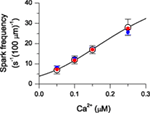Figure 3. Approximation of the calcium dependence of spark frequency. Open symbols with error bars represent data on permeabilized myocytes (Lukyanenko and Gyorke, 1999). The two indistinguishable lines represent the best fits by Eq. 2 with parameters shown in Tables 1, 2, and 4 for the optimal aHTG model for vCRSs composed of 1 and 10 random clusters with the size distribution shown in Figure 2. Small red and blue symbols represent spark frequency simulated using Eq. 4 for a set of 100 random vCRUs composed of 1 and 10 clusters.

The characteristics of Mg2+ binding to the RyR for vCRS models containing a different number of clusters (nclust) were very sensitive to nclust (Figures 4A,B; Table 4). The value of KMg was smaller by more than 50% when nclust was increased from 1 to 10. For nclust > 3 the optimal allosteric factor was larger than 1, as estimated previously for CRSs containing 182 RyRs (Zahradnikova et al., 2010b), implying a small negative allosteric effect of Mg2+ on RyR open probability. For nclust ≤ 3, the value of fMg was less than 1, implying a small positive allosteric effect of Mg2+ on RyR open probability. The estimate of the refractory period trp was not sensitive to changes in nclust (Figure 4C).

FIGURE 4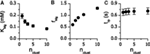Figure 4. The dependence of the fitted parameters on the number of clusters in the virtual calcium release sites. Optimized values of KMg(A), fMg (B) and trp (C) are given with the standard errors of estimate.

TABLE 4

The Mg2+-binding properties of the RyR had a significant impact on RyR open probability in the whole range of cytosolic Ca2+, as documented in Figure 5. Here the simulated calcium dependence of open probability is shown superimposed on the published experimental data obtained in the presence of 3 mM ATP and defined concentrations of free Mg2+ (Zahradnikova et al., 2003). The lower fMg and the higher KMg values at nclust of 1 and 2 led to a significantly weaker inhibition of RyR open probability than experimentally observed, especially at higher [Mg2+]. The best correspondence between data and simulation occurred for nclust = 3–10.

FIGURE 5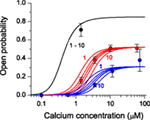Figure 5. Approximation of the calcium dependence of RyR open probability at different cytosolic Mg2+ concentrations. Lines depict theoretical open probability of RyRs with parameter values (Tables 2 and 4) optimized to fit the calcium dependence of spark frequency for vCRSs composed of 1, 2, 3, 5, and 10 clusters in the absence (black) and in the presence of 0.6 (red, left to right for increasing nclust) or 0.9 mM Mg2+ (blue, left to right for increasing nclust).

### Distribution of Calcium Release Flux

The distribution of spark calcium release flux was calculated for all simulated vCRSs using Eq. 5. When vCRSs were composed of a single multi-cluster vCRU, it was assumed that after opening of the first RyR in the vCRS, all remaining RyRs in the vCRS have the same probability pA of becoming open. When the vCRSs were composed of several single-cluster vCRUs, it was assumed that after opening of the first RyR in one vCRU, all remaining RyRs in that vCRU have the same probability pA of becoming open, while the RyRs in the remaining vCRUs have the resting probability of becoming open, i.e., they are not affected by the RyR opening in the other vCRUs of the same vCRS. Since under all tested conditions the resting open probability of the RyR was ∼1 × 10−4, the probability that a resting RyR from another cluster of the same vCRS will open during the spark was less than 0.0035, which would result in a 0.35% excess of RyR openings, less than the estimated error of approximation of the data (Wang et al., 2004) by the best model (0.95%). Therefore the contribution of resting RyR openings to the distributions of calcium release flux was not taken into account.

The activation probability pA for each set of vCRSs with a given nclust was estimated by approximating the distribution of nO (Eq. 5) to the experimental data published by Wang et al. (2004). The optimal distributions are shown in Figure 6 together with the experimental distribution. The values of pA providing the optimal fit and the resulting average numbers of open RyRs per spark are given in Table 5. For vCRSs composed of a single multi-cluster vCRU (Figure 6A), it is apparent that for low nclust of 1 or 2 the theoretical distributions did not describe the data adequately (χ2 test, p < 0.001). A satisfactory description of the data was obtained for nclust = 3–10 (χ2 test, p > 0.1). If the vCRS was composed of a set of single-cluster, non-interacting vCRUs, the theoretical distributions of the spark calcium release flux did not depend on nclust (Figure 6B) and were not adequate (χ2 test, p < 0.001).

FIGURE 6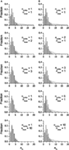Figure 6. Approximation of the distribution of calcium release fluxes with the theoretical distribution of the number of open RyRs in sparks. Black bars represent the probability distribution of spark fluxes recorded under control conditions (Wang et al., 2004, their Figure 2) expressed in units of the elementary quanta of calcium release flux; white bars represent the probability distribution of the number, nO, of RyRs open during spark, calculated using Eq. 2 for sets of simulated vCRUs formed of (top to bottom) 1, 2, 3, 5, and 10 random clusters with size distribution from Figure 2, respectively. (A) vCRSs composed of one single- or multicluster vCRU, (B) vCRSs composed of one or more single-cluster vCRUs.

TABLE 5

### The Rate of Mg2+ Dissociation from the Activation Sites

If the clusters in the vCRS formed a single vCRU, the probability of recruitment of additional RyRs after activation of the first RyR (pA) decreased with increasing nclust. We tested the extent to which these changes were due to the changes in the dissociation of Mg2+ from the activation sites. The estimated values of pA (Figure 7A) were converted into values of probability of Mg2+ dissociation from the activation site during the spark, pdis (Figure 7B), using the procedure described in the Supplemental data of Zahradnikova et al. (2010b). These were further converted to Mg2+ dissociation rates (Figure 7C), assuming that Mg2+ dissociation has to occur not later than at the time to the peak of the spark (6.67 ms, Eq. 6). The values of all three parameters decreased with nclust (circles); the models that did not correspond to the observed distributions of calcium release flux (Figure 6A) provided considerably higher values (open symbols) than the accepted models (crossed symbols). If the clusters in the vCRS formed separate vCRUs, the probability of recruitment of additional RyRs into sparks after activation of the first RyR did not change considerably with nclust (squares in Figure 7). However, neither of these models corresponded to the experimental distributions of calcium release flux (Figure 6B).

FIGURE 7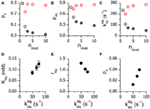Figure 7. The dependence of RyR kinetic parameters on the cluster composition of vCRSs. (A–C) The dependence of pA, pdis, and $KdisMg$on the number of clusters in the vCRS. Circles: vCRSs composed of a single multi-cluster vCRU. Squares: vCRSs composed of several single-cluster vCRUs. Crossed symbols: values from models with nclust ≥ 3 and nvCRU = 1 that match the experimental data (Figure 6). (D–F) The dependence of KMg, fMg, and pA on $KdisMg$ for vCRUs with different number of clusters.

Additionally, variation of the number of clusters present in one vCRS requested changes in the calcium dependence of RyR activation to follow the calcium dependence of RyR open probability at different Mg2+ concentrations (Figure 5). Specifically, with increasing number of clusters per vCRS, the RyRs had to undergo stronger inhibition by Mg2+ at their activation sites (smaller KMg and larger fMg). The extent, to which the changes in KMg, fMg, and pA are due to changes of $kdisMg,$ is examined in Figures 7D–F for vCRSs that were accepted by the χ2 test. The increase in $kdisMg$translates into a proportional increase of KMg and a decrease of fMg. The value of pA is very steeply dependent on $kdisMg.$

## Discussion

This study reveals that not only the gating of RyR channels, but also their organization into release sites plays a role in the cardiac mechanism of excitation-contraction coupling. This would be difficult to reveal experimentally.

The relationships between the organization of RyRs into calcium release sites and the properties of calcium sparks were explored using extensions of our previously described models of calcium spark and RyR gating (see Materials and Methods). The results have shown that for a range of calcium release site sizes $(n̄RyR=42.3-141;ñRyR=36-136.5)$ and size distributions, a model of calcium spark formation based on independent RyRs provides a quantitative description of the calcium dependence of calcium spark frequency (Figure 3). The two alternative hypotheses, that the confocally resolved volume comprises either a single vCRU containing three or more clusters, or several small vCRUs containing one cluster each, provided identical results, since all RyRs present in the confocally resolved volume are indistinguishable under resting conditions.

Moreover, the quantal distribution of spark calcium release flux in these CRUs is in a good agreement with the experimental data (Figure 6), if the RyR clusters in the calcium release sites are organized into a single CRU, that is, all RyRs of the CRS experience the same conditions modulating their steady-state open probability and probability of activation.

### The Relation between CRS Distribution, Calcium Spark Frequency and RyR Open Probability

The parameters of Mg2+ interaction with RyR channels (KMg and fMg) were optimized for different sets of vCRS sizes. A satisfactory fit of calcium spark frequency could be obtained for all vCRSs at the expense of substantial changes in the parameters KMg and fMg (Table 4).

The Ca2+ dependence of open probability of RyRs that provided the correct calcium dependence of calcium spark frequency was compared with experimental data at different Mg2+ concentrations (Figure 5). The simulated inhibitory effect of Mg2+ on the calcium dependence of RyR open probability was in better accordance with the experimental observations for larger vCRS sizes (nclust × nvCRU ≥ 3). For smaller vCRSs, the correct calcium dependence of calcium spark frequency could be obtained only if RyRs inhibition by Mg2+ at the RyR activation sites was weaker (KMg = 150–190 μM) than experimentally estimated (61–100 μM; Gusev and Niggli, 2008; Zahradnikova et al., 2010b; Tencerova et al., 2012) except for Zahradnikova et al. (2003; 250 ± 150 μM).

### Quantal Distribution of Calcium Release Flux

Unequivocal results regarding the composition of vCRSs were provided by analysis of the quantal distribution of calcium release flux in evoked calcium sparks. The theoretical predictions of the models corresponded with experimental data (Wang et al., 2004) only for vCRSs consisting of a single larger vCRU (nclust > 3). For vCRSs consisting of a single smaller vCRU (nclust = 1, 2), and for vCRSs composed of up to ten small vCRUs (nclust = 1), there was an excess of predicted sparks with high calcium release flux (nO > 7) and a deficit of the predicted sparks with average calcium release flux (nO = 2–3), which resulted in unacceptably high deviation of the model from the data. Since independent recruitment of RyRs after commencement of the spark, indicated by the binomial distribution of their calcium release flux, requires their equal access to the calcium trigger, all clusters in the vCRS had to communicate via the shared cytosolic/luminal environment, i.e., to consist of a single multi-cluster vCRU.

### The Rates of Mg2+ Dissociation from the RyR

The rate of Mg2+ dissociation obtained by optimizing the quantal distribution of calcium release flux was strongly dependent on the assumed vCRS size, and ranged from 48 to 224 s−1.A satisfactory accordance with experimental data was obtained only for vCRS size of 3–10 clusters, yielding $kdisMg=48-74s-1.$

These values are comparable with or higher than the rates of dissociation of Mg2+ from the Mg2+-parvalbumin complex (4–33 s-1; Permyakov et al., 1987) or from its complex with troponin-C or with the C-terminal fragment of troponin-C (5 and 0.7 s−1; Rosenfeld and Taylor, 1985). At the same time, they are somewhat lower than the rates of Mg2+ dissociation from ATP (150 s−1; Baylor and Hollingworth, 1998). A relatively low value of $kdisMg$ may also be inferred from the strong effect of low Mg2+ concentrations on activation of RyR channels by brief Ca2+ stimuli (Zahradnikova et al., 2003). Therefore, the values of $kdisMg$estimated for nclust of 3–10 may be considered plausible.

## Conclusion

The modeling of the calcium release sites showed that RyRs with gating characteristics conforming to their behavior in lipid bilayers, and organized in clusters with size distribution revealed by experiments, provide a very satisfactory description of calcium spark properties in both, spontaneous and triggered sparks, collected in independent experiments. According to the best models, RyR clusters associate into CRUs composed of at least 3 clusters and containing at least 40 RyRs on average, which share the same dyadic space and environmental conditions. Moreover, these results reconcile the observed small cluster size and somewhat irregular placement of RyRs in the release sites with the previous estimates of the amount of RyR protein (Bers and Stiffel, 1993), volume density of calcium release sites (Chen-Izu et al., 2006), and the extent of calcium release in rat cardiac myocytes (Zahradnikova et al., 2010b).

## Conflict of Interest Statement

The authors declare that the research was conducted in the absence of any commercial or financial relationships that could be construed as a potential conflict of interest.

## Acknowledgments

We would like to thank M. Novotová and A. Zahradníková, Jr. for inspiring discussions. Supported by grants APVV-0721-10, APVV-0628-10, and VEGA 2/0190/10, 2/0917/11 and 2/0116/12.

## References

Asghari, P., Scriven, D. R., Hoskins, J., Fameli, N., Van Breemen, C., and Moore, E. D. (2012). The structure and functioning of the couplon in the mammalian cardiomyocyte. Protoplasma 249(Suppl. 1), S31–S38.

Baddeley, D., Jayasinghe, I. D., Lam, L., Rossberger, S., Cannell, M. B., and Soeller, C. (2009). Optical single-channel resolution imaging of the ryanodine receptor distribution in rat cardiac myocytes. Proc. Natl. Acad. Sci. U.S.A. 106, 22275–22280.

Baylor, S. M., and Hollingworth, S. (1998). Model of sarcomeric Ca2+ movements, including ATP Ca2+ binding and diffusion, during activation of frog skeletal muscle. J. Gen. Physiol. 112, 297–316.

Bers, D. M., and Stiffel, V. M. (1993). Ratio of ryanodine to dihydropyridine receptors in cardiac and skeletal muscle and implications for E-C coupling. Am. J. Physiol. Cell Physiol. 264, C1587–C1593.

Bridge, J. H., Ershler, P. R., and Cannell, M. B. (1999). Properties of Ca2+ sparks evoked by action potentials in mouse ventricular myocytes. J. Physiol. (Lond.) 518, 469–478.

Cannell, M. B., Cheng, H., and Lederer, W. J. (1994). Spatial non-uniformities in [Ca2+]i during excitation-contraction coupling in cardiac myocytes. Biophys. J. 67, 1942–1956.

Cheng, H., Lederer, W. J., and Cannell, M. B. (1993). Calcium sparks: elementary events underlying excitation-contraction coupling in heart muscle. Science 262, 740–744.

Chen-Izu, Y., Mcculle, S. L., Ward, C. W., Soeller, C., Allen, B. M., Rabang, C., Cannell, M. B., Balke, C. W., and Izu, L. T. (2006). Three-dimensional distribution of ryanodine receptor clusters in cardiac myocytes. Biophys. J. 91, 1–13.

Franzini-Armstrong, C., Protasi, F., and Ramesh, V. (1999). Shape, size, and distribution of Ca(2+) release units and couplons in skeletal and cardiac muscles. Biophys. J. 77, 1528–1539.

Gusev, K., and Niggli, E. (2008). Modulation of the local SR Ca2+ release by intracellular Mg2+ in cardiac myocytes. J. Gen. Physiol. 132, 721–730.

Hayashi, T., Martone, M. E., Yu, Z., Thor, A., Doi, M., Holst, M. J., Ellisman, M. H., and Hoshijima, M. (2009). Three-dimensional electron microscopy reveals new details of membrane systems for Ca2+ signaling in the heart. J. Cell. Sci. 122, 1005–1013.

Landau, R. H., and Páez, M. J. (1997). Computational Physics. New York, NY: John Wiley & Sons.

Lukyanenko, V., Gyorke, I., Subramanian, S., Smirnov, A., Wiesner, T. F., and Gyorke, S. (2000). Inhibition of Ca(2+) sparks by ruthenium red in permeabilized rat ventricular myocytes. Biophys. J. 79, 1273–1284.

Lukyanenko, V., and Gyorke, S. (1999). Ca2+ sparks and Ca2+ waves in saponin-permeabilized rat ventricular myocytes. J. Physiol. (Lond.) 521, 575–585.

Lukyanenko, V., Viatchenko-Karpinski, S., Smirnov, A., Wiesner, T. F., and Gyorke, S. (2001). Dynamic regulation of sarcoplasmic reticulum Ca(2+) content and release by luminal Ca(2+)-sensitive leak in rat ventricular myocytes. Biophys. J. 81, 785–798.

Maier, L. S., Zhang, T., Chen, L., Desantiago, J., Brown, J. H., and Bers, D. M. (2003). Transgenic CaMKIIdeltaC overexpression uniquely alters cardiac myocyte Ca2+ handling: reduced SR Ca2+ load and activated SR Ca2+ release. Circ. Res. 92, 904–911.

Permyakov, E. A., Ostrovsky, A. V., and Kalinichenko, L. P. (1987). Stopped-flow kinetic studies of Ca(II) and Mg(II) dissociation in cod parvalbumin and bovine alpha-lactalbumin. Biophys. Chem. 28, 225–233.

Press, W. H., Flannery, B. P., Teukolsky, S. A., and Vetterling, W. T. (1992). Numerical Recipes in C, 2nd Edn. Cambridge: Cambridge University Press.

Rosenfeld, S. S., and Taylor, E. W. (1985). Kinetic studies of calcium and magnesium binding to troponin C. J. Biol. Chem. 260, 242–251.

Shkryl, V. M., Blatter, L. A., and Rios, E. (2012). Properties of Ca2+ sparks revealed by four-dimensional confocal imaging of cardiac muscle. J. Gen. Physiol. 139, 189–207.

Soeller, C., Crossman, D., Gilbert, R., and Cannell, M. B. (2007). Analysis of ryanodine receptor clusters in rat and human cardiac myocytes. Proc. Natl. Acad. Sci. U.S.A. 104, 14958–14963.

Sun, X. H., Protasi, F., Takahashi, M., Takeshima, H., Ferguson, D. G., and Franzini-Armstrong, C. (1995). Molecular architecture of membranes involved in excitation-contraction coupling of cardiac muscle. J. Cell Biol. 129, 659–671.

Tencerova, B., Zahradnikova, A., Gaburjakova, J., and Gaburjakova, M. (2012). Luminal Ca controls activation of the cardiac ryanodine receptor by ATP. J. Gen. Physiol. 140, 93–108.

Wang, S. Q., Stern, M. D., Rios, E., and Cheng, H. (2004). The quantal nature of Ca2+ sparks and in situ operation of the ryanodine receptor array in cardiac cells. Proc. Natl. Acad. Sci. U.S.A. 101, 3979–3984.

Zahradnik, I., Gyorke, S., and Zahradnikova, A. (2005). Calcium activation of ryanodine receptor channels – reconciling RyR gating models with tetrameric channel structure. J. Gen. Physiol. 126, 515–527.

Zahradnikova, A., Dura, M., Gyorke, I., Escobar, A. L., Zahradnik, I., and Gyorke, S. (2003). Regulation of dynamic behavior of cardiac ryanodine receptor by Mg2+ under simulated physiological conditions. Am. J. Physiol. Cell Physiol. 285, C1059–C1070.

Zahradnikova, A., Gaburjakova, M., Bridge, J. H., and Zahradnik, I. (2010a). Challenging quantal calcium signaling in cardiac myocytes. J. Gen. Physiol. 136, 581–583.

CrossRef Full Text

Zahradnikova, A., Valent, I., and Zahradnik, I. (2010b). Frequency and calcium release flux of calcium sparks in rat cardiac myocytes: a relation to RyR2 gating. J. Gen. Physiol. 136, 101–116.

CrossRef Full Text

Zahradnikova, A., Zahradnik, I., Gyorke, I., and Gyorke, S. (1999). Rapid activation of the cardiac ryanodine receptor by submillisecond calcium stimuli. J. Gen. Physiol. 114, 787–798.

Keywords: excitation-contraction coupling, cardiac myocyte, local calcium signaling, ryanodine receptors, calcium sparks

Citation: Zahradníková A and Zahradník I (2012) Construction of calcium release sites in cardiac myocytes. Front. Physio. 3:322. doi: 10.3389/fphys.2012.00322

Received: 18 April 2012; Accepted: 23 July 2012;
Published online: 20 August 2012.

Edited by:

Eric A. Sobie, Mount Sinai School of Medicine, USA

Reviewed by:

Silvina Ponce Dawson, Universidad de Buenos Aires, Argentina
Jose Luis Puglisi, University of California Davis, USA